## There are 35 times as many students at Wow University as teachers. When all the students and teachers are seated in the 8544 seat audi

Question

There are 35 times as many students at Wow University as teachers. When all the students and
teachers are seated in the 8544 seat auditorium, 12 seats are empty. How many students attend
Wow University?

in progress 0
2 months 2021-07-28T08:17:36+00:00 1 Answers 1 views 0

1. Given:

There are 35 times as many students at Wow University as teachers.

When all the students and teachers are seated in the 8544 seat auditorium, 12 seats are empty.

To find:

The total number of students.

Solution:

Let x be the number of teachers at Wow University. So, the number of student is :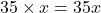When all the students and teachers are seated in the 8544 seat auditorium, 12 seats are empty.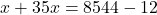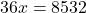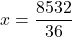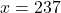The number of total students is: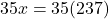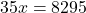Therefore, the total number of students is 8295.